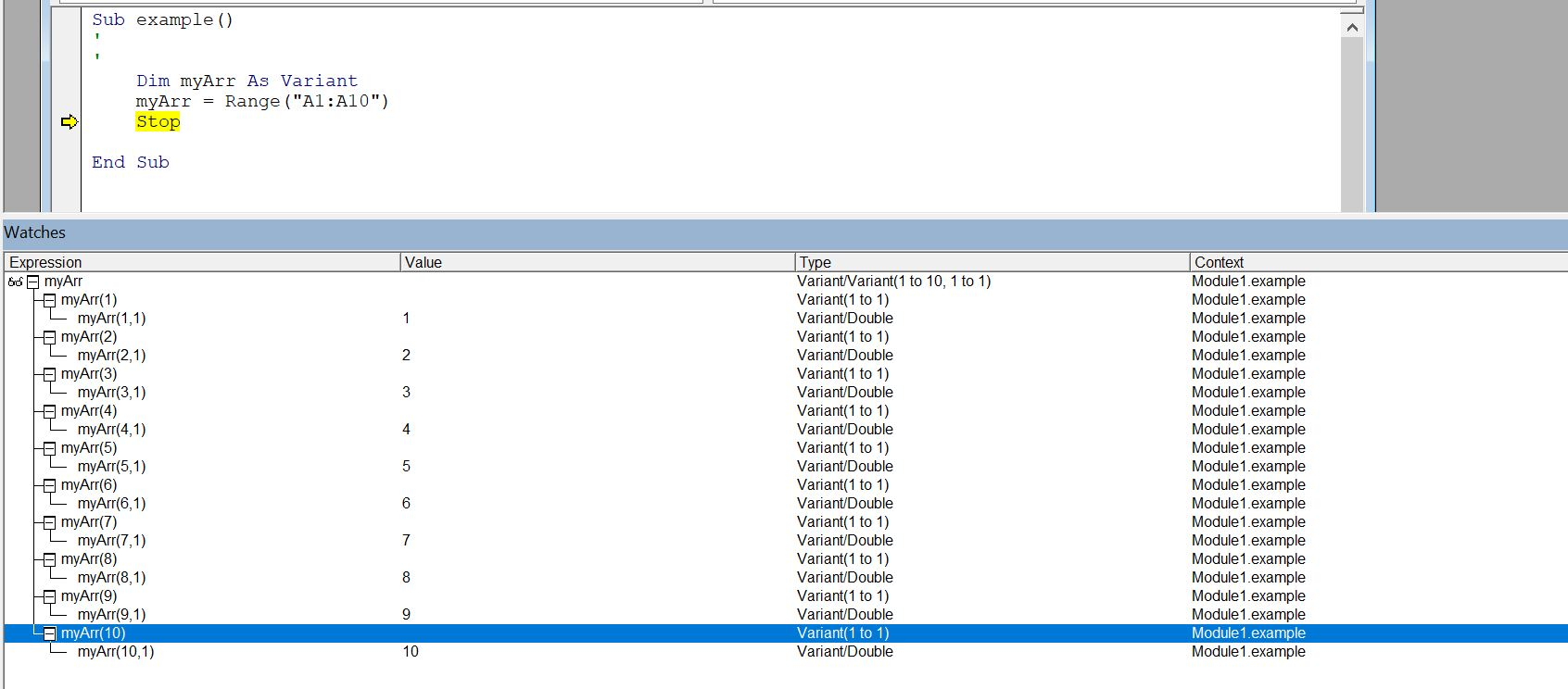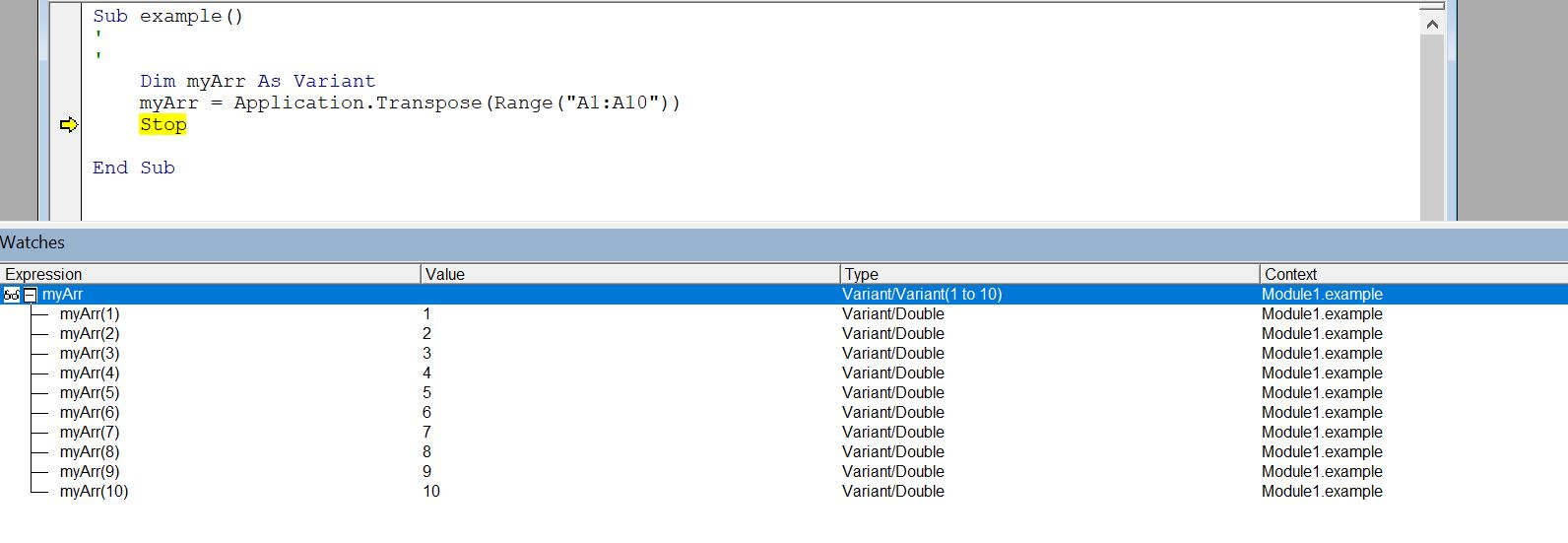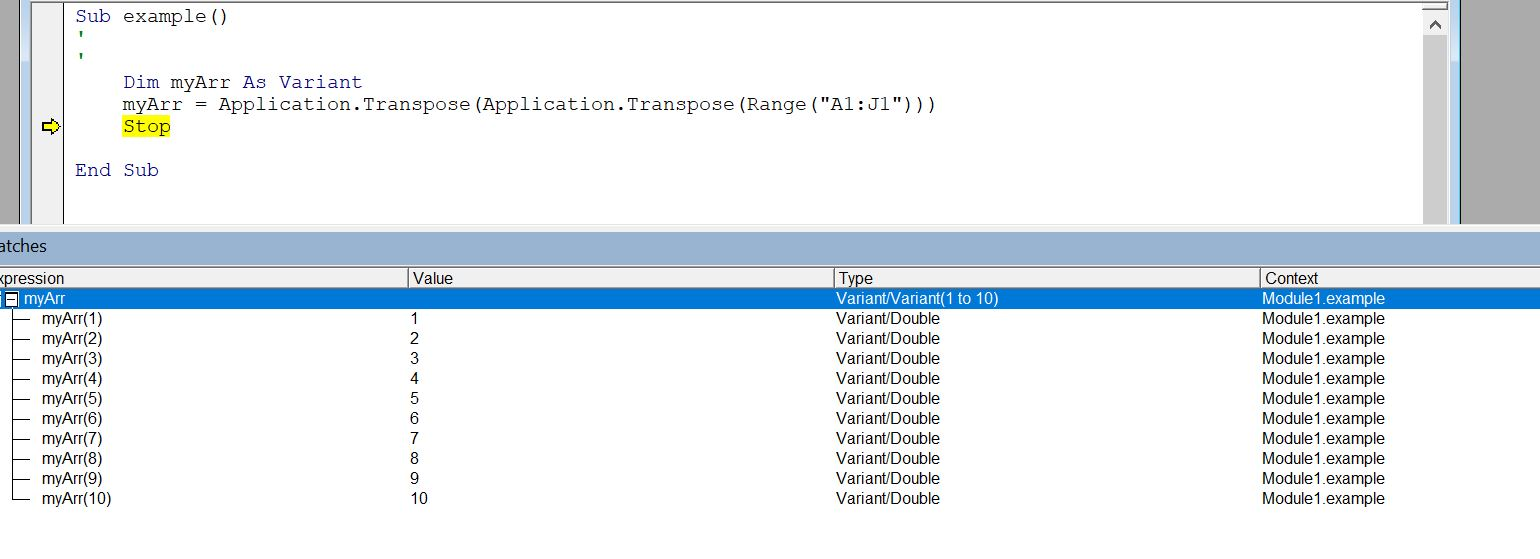# Create an Array From a Specific Range in Excel Using VBA

Bilal Shahid Sep 23, 2022 VBA VBA Array

This tutorial educates us about arrays in VBA and demonstrates how we can create an array using the specified range in MS Excel using VBA.

Before moving toward the main topic, learning about arrays in VBA is essential. Let’s start with that.

## Arrays in VBA

The array is a collection of similar objects that we can access with the help of an index. It is pretty easy to define an array in VBA. We can declare two types of an array in VBA - `static array` and `dynamic array`.

A `static array` is a fixed-size array that can only store the number of values it has been defined. See the following example where we declare an array of `string` data types with size `7`:

`````` Dim arr(7) As String
``````

A `dynamic array` does not have a defined size, and we can extend it along the program. We can declare it using either of the two statements mentioned below:

``````Dim arr() As String
``````

OR

``````Dim arr As Variant
``````

It is quite easy to assign values to an array in VBA. For a `static array`, we can use the following statement to assign values:

``````arr (index) = Value
``````

Initially, the `dynamic array` is not declared with size; therefore, to assign values to a `dynamic array`, redeclare it with the size and then assign any value.

``````ReDim arr(size)
arr (index) = value
``````

Now we know enough about arrays in VBA, let’s learn how to create an array with the specified range in Microsoft Excel using VBA.

## Create an Array With a Specific Cell Range

The section written above describes how to declare a simple array. However, it does not mention creating an array that contains values from a specific cell range in Microsoft Excel.

To assign an array with the cell values, we can use the following code statement:

``````Dim arr As Variant
arr= Range("A1:A10").Value
``````

The `arr` is declared as a `dynamic array` so it can accept the cell range that is assigned to it. The code statement executes perfectly but creates a two-dimensional array over the cell range `A1:A10`. We can see it in the image below:Note: The array index starts from `1` instead of `0`.

It can be difficult for users, especially beginners, to work with a two-dimensional array.

Therefore, it is essential to figure out a solution that can convert the two-dimensional array into a one-dimensional array because it is always easier to work with a single-dimensional array.

### Convert the 2D Array Into a 1D Array

There are two scenarios when converting the two-dimensional array into a single-dimensional array. One scenario is when the cell range is that of a column. The other scenario is when the cell range is that of a row.

#### Column Cell Range

When you want to store the values of a column in a single-dimensional array, you can use the `Application.Transpose()` function on the `Range()` function.

It will return a single-dimensional array containing the cell values from the column range mentioned in the `Range()` function. For that, we can use the following code fence:

``````Dim myArr As Variant
myArr = Application.Transpose(Range("A1:A10"))
``````

On execution, the code statements produce the following results:#### Row Cell Range

The `Application.Transpose()` function needs to be used twice on the `Range()` function when you want to store the values of a row in a single-dimensional array.

The resulting array will be a single-dimensional array that contains the cell values from the row range mentioned in the `Range()` function. To do that, we can use the following code block:

``````Dim myArr As Variant
myArr = Application.Transpose(Application.Transpose(Range("A1:J1")))
``````

On execution, the code statements produce the following results:Note: We notice that the array starts from index `1` instead of index `0` in both scenarios. The `Application.Transpose()` function does not alter the indexes of the array.

It is also important to note that The newer versions of Microsoft Excel use a modified version of the `Application.Transpose()` function.

The `Application.WorksheetFunction.Transpose()` is used in the newer versions of Microsoft Excel as an alternative to the `Application.Transpose()` function.

Author: Bilal Shahid

Hello, I am Bilal, a research enthusiast who tends to break and make code from scratch. I dwell deep into the latest issues faced by the developer community and provide answers and different solutions. Apart from that, I am just another normal developer with a laptop, a mug of coffee, some biscuits and a thick spectacle!

GitHub

## Related Article - VBA Array

• Use for Each on an Array Using VBA
• Return Array From Function in VBA
• Get the Array Length in VBA
• Declare and Initialize String Array in VBA
• Sort the Elements of an Array and Arraylist in VBA